# What is NEWTON'S LAW OF GRAVITATION - Concept with example.

NEWTON'S LAW OF GRAVITATION :

It states that every body in the universe attracts every other body with a force which is directly proportional to the product of their masses and is inversely proportional to the square of the distance between them.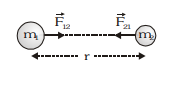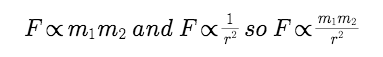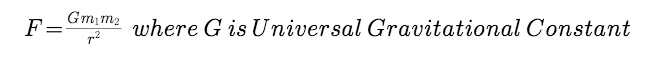Vector form of Newton's law of Gravitation :

let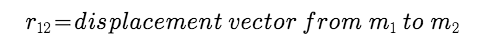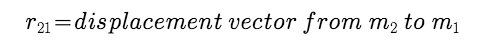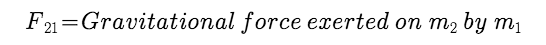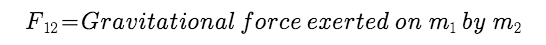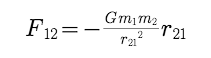Negative sign shows that :

(i) The direction of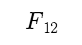is opposite to that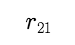(ii) The gravitational force is attractive in nature

The gravitational force between two bodies are equal in magnitude and opposite in direction.

Example :

1.Two particles of masses 1 kg and 2 kg are placed at a separation of 50 cm. Assuming that the only forces acting on the particles are their mutual gravitation, find the initial acceleration of heavier particle.

Solution :

Force exerted by one particle on another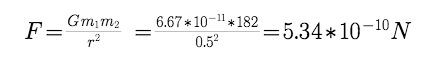Acceleration of heavier particle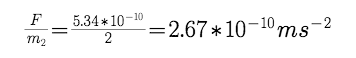Example 2:

Two stationary particles of masses M1 and M2 are at a distance 'd' apart. A third particle lying on the line joining the particles, experiences no resultant gravitational forces. What is the distance of this particle from M1 .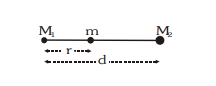The force on m towards M1 is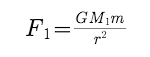The force on m towards M2 is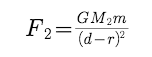According to question net force on m is zero i.e. F1 = F2Example :

Two particles of equal mass (m) each move in a circle of radius (r) under the action of their mutual gravitational attraction. Find the speed of each particle?

Solution :

For motion of particle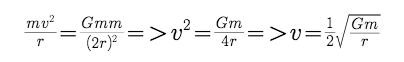Post By : Preeti Rai 15 Mar, 2019 1801 views Physics